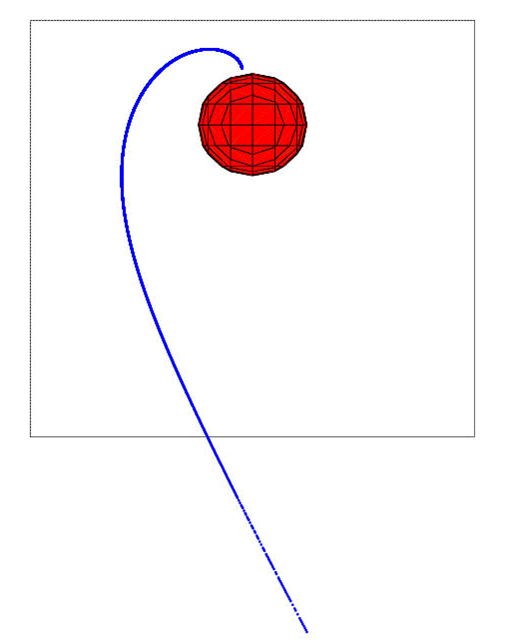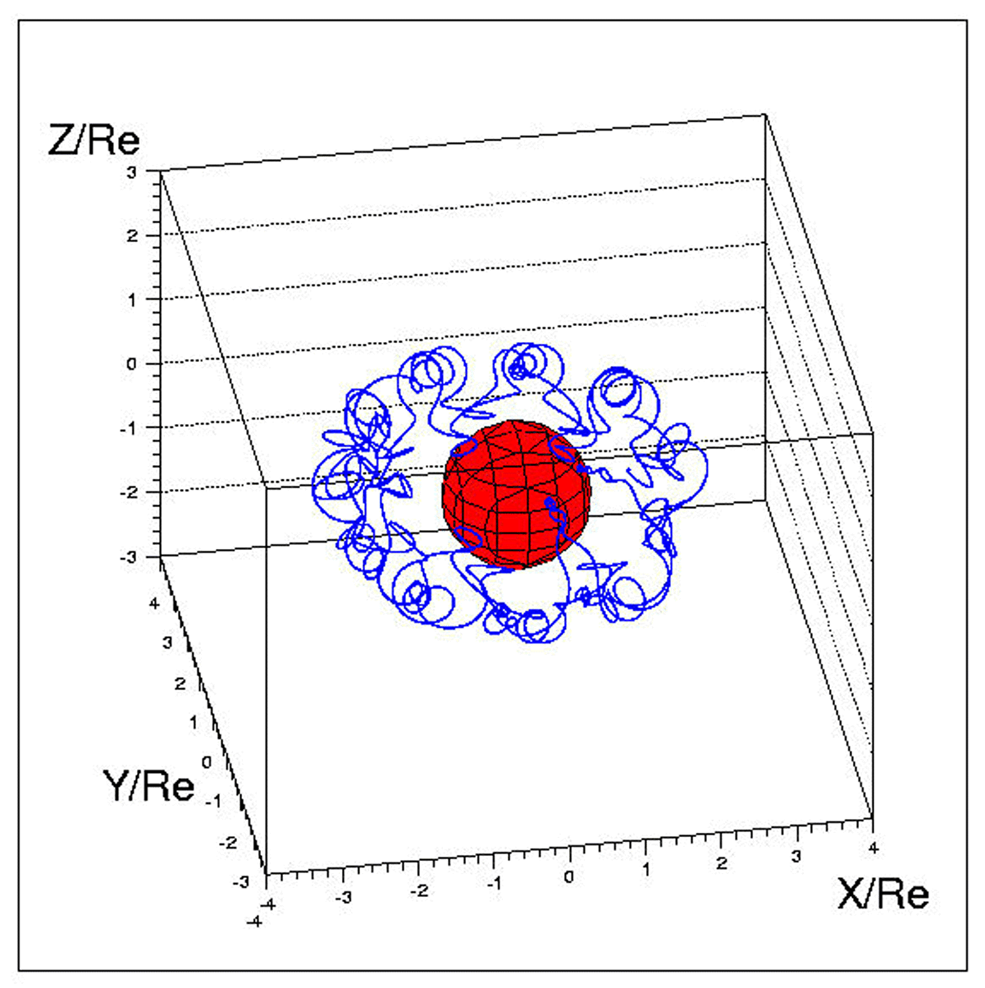The geomagnetic rigidity cut-off discriminates between primary cosmic rays (coming form outside the magnetosphere) and secondary particles (trapped or generated inside the magnetosphere). Another method adopted to separate these two populations is to do back-tracing for each event, i.e. the reconstruction of the particle trajectory back in time from the detection position back to the edge of magnetosphere (for primaries), using a numerical code that includes accurate description of the geomagnetic field (see e.g. Bobik et al. 2005).

The magnetosphere models are developed referring to the Tsyganenko's studies. These models add to the well known internal magnetic field also an external magnetic field, which is strongly asymmetric, with a tail that extends in opposite direction to the Sun. Having a good description of the magnetic field, the particle back-tracing from the detection position to the magnetosphere border could be done using experimental data, such as solar pressure, coming from satellites around the Earth. The trajectory of the particle is reconstructed, in a unique way, according to its rigidity, arrival position and direction. For a better explanation of the back-tracing model adopted see e.g., Bobik et al. 2005 and Bobik et al.2006.

The code uses, as input parameters, the properties of the particle: mass, charge, rigidity, position (i.e., geographic coordinates) and incoming direction (reversed for back-tracing), date and time. We used different magnetic field models: IGRF as internal magnetic field, T96 or TS05 as external magnetic field. The particle back-tracing gives us information related to the last point (position and direction) on the magnetosphere border (for primary particles), the time and length of the trajectory.

Before starting the reconstruction of the trajectory, the existence of the external parameters like the solar wind ram pressure $$p_{dyn}$$, Dst-index and transverse components (B$$_y$$ and B$$_z$$) of the interplanetary magnetic field at each given date are checked. If these parameters are available, they must to be also inside the following limits: $$p_{dyn}$$ between 0.5 and 10 nPa, Dst-index between -100 and 20 nT, IMF-B$$_y$$ and IMF-B$$_z$$ between -10 and 10 nT. For the TS05 models other six parameters are required; ($$W_1,...,W_6$$) are functions of solar wind density $$N_{sw}$$, speed $$V_{sw}$$ and the southward interplanetary magnetic field B$$_z$$. After the checks on the parameters it is possible to start the reconstruction of the particle trajectory back in time.Figure 1: Example of cosmic ray backtracing, trajectory reconstruction, for a primary particle (left) and a trapped particle (right).

Hits: 4404